DEVELOPMENT AND EXPERIMENTAL VALIDATION OF A THERMOELECTRIC TEST BENCH FOR LABORATORY LESSONS

# DEVELOPMENT AND EXPERIMENTAL VALIDATION OF A THERMOELECTRIC TEST BENCH FOR LABORATORY LESSONS

 Antonio Rodríguez García, David Astrain Ulibarrena, Álvaro Martínez Echeverri, Patricia Aranguren Garacochea, Gurutze Pérez Artieda Universidad Pública de Navarra, ETSIIT, Dpto. de Ingeniería Mecánica, Energética y de Materiales Spain antonio.rodriguez@unavarra.es

Accepted September 2013

Abstract

The refrigeration process reduces the temperature of a space or a given volume while the power generation process employs a source of thermal energy to generate electrical power. Because of the importance of these two processes, training of engineers in this area is of great interest. In engineering courses it is normally studied the vapor compression and absorption refrigeration, and power generation systems such as gas turbine and steam turbine. Another type of cooling and generation less studied within the engineering curriculum, having a great interest, it is cooling and thermal generation based on Peltier and Seebeck effects.

The theoretical concepts are useful, but students have difficulties understanding the physical meaning of their possible applications. Providing students with tools to test and apply the theory in real applications, will lead to a better understanding of the subject. Engineers must have strong theoretical, computational and also experimental skills.

A prototype test bench has been built and experimentally validated to perform practical lessons of thermoelectric generation and refrigeration. Using this prototype students learn the most effective way of cooling systems and thermal power generation as well as basic concepts associated with thermoelectricity. It has been proven that students learn the process of data acquisition, and the technology used in thermoelectric devices.

These practical lessons are implemented for a 60 people group of students in the development of subject of Thermodynamic including in the Degree in Engineering in Industrial Technologies of Public University of Navarra.

Keywordspractical lessons, thermoelectric cooling and thermoelectric generation

----------

1 INTRODUCTION

Building and validating the prototype practices of the test bench to study the thermoelectric generation and cooling is the motivation of this work. The main contribution of the teaching practices is the better understanding of the students of the phenomena associated with the topic of study, based on physical concepts.

In engineering courses students begin acquiring knowledge of mathematics, chemistry and physics. Then they continue with the development of skills for solving problems in basic sciences such as mechanics, materials science, fluid mechanics and thermodynamics. Finally they obtain more specific knowledge of refrigeration and power generation in more applied subjects such as thermal engineering.

Cooling systems normally studied in engineering courses are vapor compression systems, used in household refrigerators in most air conditioners, and absorption systems used for large installations where electricity is limited and / or there is a large heat flow. Another type of cooling, less studied within the engineering curriculum, having a prospective future, as seen in (Vián & Astrain, 2009) and (Rodríguez, Vián & Astrain, 2009), is thermoelectric cooling based on the Peltier effect.

In the case of electricity generation, power cycles of both gas (Brayton cycle) and steam (Rankine cycle) are the most studied in the engineering curricula. Another type of power generation system is the thermoelectric generation. Given the current energy situation and the environmental problems, it is becoming more necessary to optimize systems for power generation and find new sources of alternative energy, cleaner and more sustainable. In this regard, researchers have put their perspectives on the use of the so-called residual or waste energy, as an energy resource, untapped byproduct obtained from different processes, which in industrialized countries represents about 40% of the primary energy consumed. Thermoelectric generators are one of the best options to take advantage of residual energy sources, as shown in (Astrain, Vián, Martínez & Rodríguez, 2010).

If two different materials are joined together (this is called a thermocouple) and one of the joints has higher temperature than the other one, a voltage difference appears making current circulate in between the cold and hot joints. This phenomenon is known as the Seebeck effect. When a current circulates through a system composed by different materials, the opposite effect occurs, in this case in one of the joints heat is absorbed while in the other one heat is thrown out. This phenomenon’s name is Peltier effect.

To analytically compute the heat fluxes and the temperatures that take part in a thermoelectric system, it is necessary to solve for the heat transmission equation in the interior of the module as well as the heat fluxes, Qp, QJ and Qτ corresponding equations due to Peltier, Joule and Thomson effects.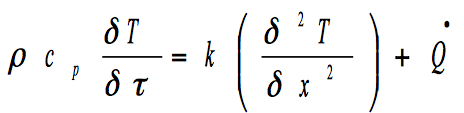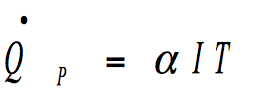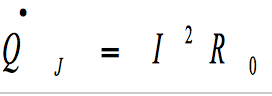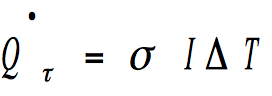(1) (2) (3) (4)

The system obtained is a nonlinear system with second order derivatives. This system cannot be solved without some simplifying hypothesis, (Rowe, 1995). In our investigation group several computational models, which allow reducing the number of simplifying hypothesis, have been developed. Thus the precision of the model increases, the accuracy of such computational model can be seeing in (Rodríguez, Astrain, Martinez, Gubía & Sorbet, 2013) and (Rodríguez et al., 2009)

The aim of this study was to build and validate a practical methodology for the student, in this way, they are able to become familiar with thermoelectric refrigeration and generation. The methodology used has been the validation of the experimental practices in which students conduct the trials and collect data for analytical calculations. Then, they should get the findings in each case, so the student gets a better understanding of the problem, instead of studying a static problem in a book. Besides, during practices the student becomes familiar with the process of data acquisition and processing, and the technology used in thermoelectric devices.

These practices will be implemented in the Public University of Navarra internship widening the new Engineering degrees at the scene of the European Higher Education Area.

The cooling and generation practices were created for students to progress in their learning sequentially. They are titled:

• Study of the temperature difference between the faces of the thermoelectric module to different supply voltages

• Study of the electric voltage generated in the thermoelectric module terminals for different temperatures between the module faces

• Calculation of the dissipator thermal resistance on the hot side of thermoelectric cooling system

• Calculation of thermoelectric cooling system COP in function of the supply voltage

• Calculation of thermoelectric cooling system COP in function of dissipator thermal resistance

• Determination of thermoelectric generation system efficiency in function of the temperature difference between the module faces.

• Determination of thermoelectric generation system efficiency in function of the dissipator thermal resistance

2 OBJECTIVES

The main objective of this work is to develop and construct an educational test bench to study, in laboratory lessons, aspects related to thermoelectric generation and cooling. In order to accomplish this aim, the following specific objectives are proposed:

• To build a first prototype of thermoelectric generation and cooling test bench.

• To teach the process of data acquisition and processing as well as the technology used in this thermoelectric devices.

• To validate this laboratory lessons comparing experimental data with analytical data, in order to make students understand that some systems are difficult to study mathematically but can be more easily studied experimentally.

3 DEVELOPMENT AND CONSTRUCTION OF AN EDUCATIONAL TEST BENCH

A refrigerator or a basic thermoelectric generator consists of a thermoelectric module, a heat source and a dissipator. Figure 1 shows a schematic of an educational test bench (developed in collaboration between the Public University of Navarre and ENERKIT thermoelectric solutions). We designed a system in which a heat flow is passed through one side of the thermoelectric module, which simulates heat flow absorbed by the system. This heat flow is a known and adjustable value. When working as a cooler the heat flow can be regulated measuring the electric power consumed by the thermoelectric modules, being able to measure the temperature reached on both sides of the module, and the room temperature. When working as a generator the student measures the temperature reached on both sides of the module, the room temperature and the electric power generated (voltage and current).

To achieve these objectives, we need basic elements:

• A heat source (for example the heat flow dissipated by an electric resistor), in contact with one side of the thermoelectric module.

• A thermoelectric module. Dimensional data and properties of the materials must be known.

• A dissipator to evacuate the heat flow.

• A temperature measuring system based on a set of temperature sensors placed in different points and connected to a conventional data acquisition system.

• Power supply to provide electrical power consumed by the resistor or the thermoelectric module in the case of thermoelectric refrigeration.

• A multimeter to measure the electric power generated, thermoelectric generation.

• Other necessary elements, insulation, conductive thermal paste, structural components and electrical connection elements.

The thermoelectric module is the core of the system. Connected to a power source, it absorbs heat flow on the cold side, being this value the cooling capacity of the system. On the contrary, when an external heat flow is supplied to the module, it can generate electric power. A thermoelectric module is composed by N pairs of semiconductor elements of equal size, connected electrically in series and thermally in parallel.

Thermal transmission processes, both on the hot side and the cold side, are of great importance in thermoelectric technology devices, since directly influences the efficiency of devices. In most applications of thermoelectric cooling, heat dissipation from the hot side of the thermoelectric module is performed by a finned dissipator and a fan, as shown in (Astrain et al., 2010) and (Astrain, 2002). It is necessary to make a study of the thermal resistance, which can be done experimentally or throughout computational programs of fluids mechanics.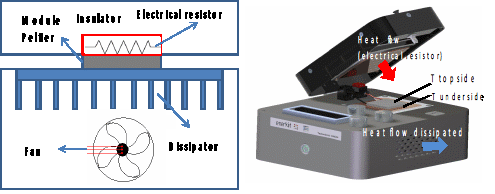Figure 1. Scheme of educational test bench for thermoelectric application

Figure 2a shows a photo of the prototype (built by ENERKIT thermoelectric solutions). This test bench allows changing the heat flow received by one side of the Peltier module, the supply voltage of the thermoelectric module and the voltage on the fan which cools down module. The test bench has a display, figure 2b, where students can take the necessary data.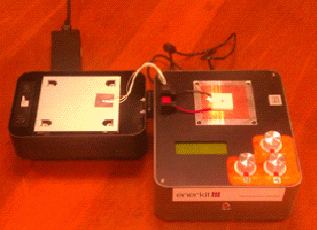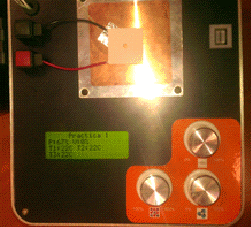Figure 2a. Test bench prototype Figure 2b. Display showing data

4 EXPERIMENTAL VALIDATION OF THE TEACHING PRACTICES IN THERMOELECTRIC APPLICATIONS

The test bench design was published by our research group in a previous work (Rodríguez, Astrain, Martínez & Garcia, 2012). In this work we showed the first prototype designed.

It is designed and built so that can perform several practices. From these practices students learn that some systems are difficult to model mathematically being easier be modeled experimentally. On the other hand they can understand better the physical problem and practice allow them to realize concepts such as energy balance, heat flux cooling, COP, electrical output and efficiency.

Refrigeration and generation practices have been created for students to progress in their learning sequentially. The designed and built test bench allows for a variety of practice due to the large number of parameters which you can work with. In our case, we have presented the validation of one of them.

These practical lessons were implemented to teach a group of 60 students during the fall semester of 2012-2013 in the subject of Thermodynamic. Lab sessions included a supervised small group of work, four or five students, having two tutored sessions of 2 hours each. The lab report had to be written in a 10 pages document. This work constituted 5% of the final grade of the course.

The methodology actions taken were:

• Previously explain in class the theoretical concepts

• Divide and assign the practices among the existing groups

• Make an example of every proposed practice.

• Supervise the proper data acquisition of the students

• Correct and grade lab reports.

As an example, practice number 3 (Calculation of the dissipator thermal resistance on the hot side of the thermoelectric cooling system) is shown.

4.1 Calculation of dissipator thermal resistance in the thermoelectric cooling system

The thermal resistance of a material represents the material's ability to resist the heat flow. The practice goal is to calculate the dissipator thermal resistance in the thermoelectric cooling system. The value of the thermal resistance depends on the dissipator´s geometry, physical properties and environmental conditions.

Thermoelectric modules generate heat flows in small areas. To prevent from overheating, it is necessary to place a dissipator on the module hot side. Without dissipators thermal resistance between the environment and the module would be so high that this would lead into a heating up of the module up to values very close to the module´s thermal limit (85 ° C). To decrease this thermal resistance a dissipator on the hot face of the module is set achieving a substantial increase in the area and therefore reducing the thermal resistances. To improve the dissipator a wind tunnel with a fan is added helping to reduce the thermal resistance of the assembly. Figure 3 presents a schematic of the existing heat fluxes in a thermoelectric refrigerator.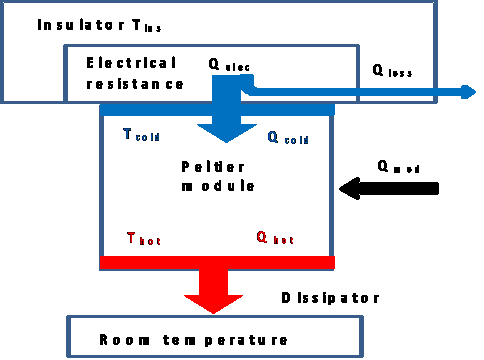Figure 3. Scheme of heat fluxes in a thermoelectric refrigerator

The thermal resistance can be estimated by analytical expressions, programs of computational fluid dynamics or experimentally. In this practice is to be calculated with semiempirical expressions convection coefficients (in order to calculate thermal resistance between the insulation and the environment) and experimental data, and will be compared with experimental values only.

In order to calculate the dissipator thermal resistance the student must complete the following steps:

Firstly, students have to set the initial conditions of the experiment.

1. Set Peltier module voltage: Vmod = 4 V.

2. Set heat flux absorbed. Velec = 4V; Qelec = 6.70 W

3. Set fan voltage Vvent = 2 V

Once the experiment is set, it is necessary to wait 15 minutes to lead time to the system to get to its thermal equilibrium and reach its steady state.

4. During this time students, using semiempirical expressions, calculate the thermal resistance of the insulation. The thermal resistance between the insulator and the environment is formed by two resistors, a conduction one and a convection resistance. Students can obtain the area of the insulation Ains =0.018 m2 and its thickness Lins=0.02 m from the test bench data sheets The thermal conductivity is obtained from the literature (Astrain, 2002) and the convection coefficient by semiempirical expressions such as (Churchill & Chu, 1975) and (Goldstein & Sparrow, 1973).

Rins-room = 65 K / W.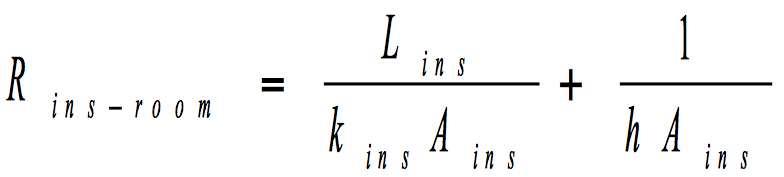(5)

Once the thermal equilibrium is achieved, temperatures are measured.

1. Measure temperatures: module cold Face Tcold = 284.5 K; module hot face Thot = 296.4 K; insulator Tins= 290.0 K; Environment Troom = 293.0 K

Calculating the thermal resistance between the insulation and the environment, and using temperature experimental data, the heat flow loss can be obtained: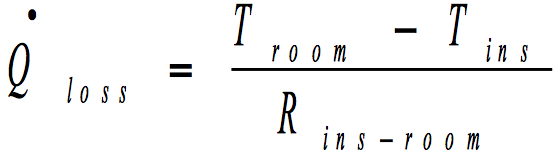(6)

Qloss = 0.046 W.

1. Applying energy balances on the electric resistor and the thermoelectric module, students can determine the heat flow evacuated from the hot side’s dissipator On the other hand, we know the value of the heat flux supplied to the system through the electrical resistance. Solving the energy balance of the electrical resistance, the heat flow introduced into the cold side is obtained. (Qcold=6.65 W). Once the heat flux introduced into the cold side estimation is known, the power consumed by the thermoelectric module is estimated (Qmod =5.09 W).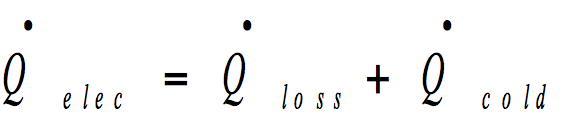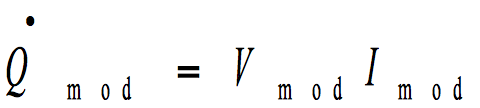(7) (8)
1. We determine the heat flow from the hot face with an energy balance in the thermoelectric module, Qhot_=_11.75 W.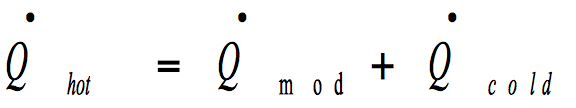(9)

Knowing the hot face and the environment temperature, and the heat flow dissipated on the hot face, the dissipator thermal resistance is determined, Rdissip = 0.57K / W.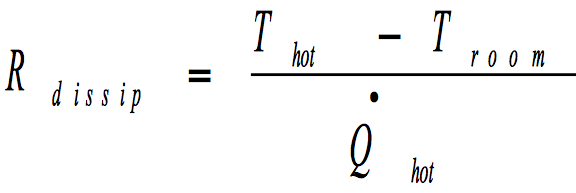(10)
1. Just utilizing experimental data implies that students need to set initial conditions. To calculate the dissipator thermal resistance experimentally we generate a heat flow until the temperature of the insulator surface is the same as the environment. Thus the loss heat flow is zero. In this way the flow of heat generated by the electric resistance is the same as the heat flow absorbed by the cold side of the thermoelectric module. These conditions were previously calculated experimentally and are given to students.

Qcold = 8.2 W.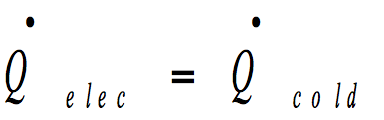(11)
1. After determining the heat flow input on the cold side, steps 5, 6 and 7 are repeated.

Qmod = 5.10 W. Qhot= 13.50 W. y Rdissip= 0.61K/W.

Students will repeat the experiments varying the power of the dissipator’s fan, thus varying the convection coefficient, in other words, the termal resistance of the dissipator.

2. The practice is repeated for different electric voltage values of fan.

The student (in the section of results and conclusions) will have to fill a table to represent graphically the dissipator thermal resistance in function of fan electric voltage. As shown in Table 1.

 Vfan (V) Rdissipator (K/W) Analytical expressions data Experimental data 4 0.57 0.61 6 0.43 0.45 8 0.35 0.38

Table 1. Dissipator termal resistance in function of fan electric voltage

5 CONCLUSIONS AND FINAL REMARKS

It has been built and validated a practical bench to study cooling and thermal generation. It has also been simulated thermoelectric refrigeration or generation. We have achieved a design that allows accessible data acquisition and has wide flexibility in thermal studies. When students work with experimental and measurement equipment they are prepared to analyze and make decisions in the labor market, using strategies that ensure the proper performance of machines and being able to suggest further changes to improve them, getting professional development.

It has been a satisfactory experience for both, the students and the teacher in charge of the practices. In this way, students can reach a new technology that is not normally seeing in their studies. The principal drawback is that the practices are developed in too big groups avoiding a proper supervision from the teacher.

ACKNOWLEDGMENTS

The authors are indebted to the Spanish Ministry of Economy and Competitiveness, and European Regional Development Fund for the economic support to this work, included in the DPI2011-24287 research project.

REFERENCES

Astrain D., (2002). Tesis Doctoral “Optimización de la Disipación de Calor en Pastillas de Efecto Peltier, Aplicación en Refrigeración Doméstica”, Departamento de Ingeniería Mecánica, Energética y Materiales. Universidad Pública de Navarra, Pamplona.

Astrain, D., Vián, J.G., Martínez, A., & Rodríguez, A. (2010). Study of the influence of heat exchangers’ thermal resistances on a thermoelectric generation system. Energy, 35, 602-610. http://dx.doi.org/10.1016/j.energy.2009.10.031

Churchill, S.W., & Chu, H.H.S. (1975). Correlating Equations for Laminar and Turbulent Free Convection from a Vertical Plane. Int. J. Heat and Mass Transfer, 18, 1323-1329. http://dx.doi.org/10.1016/0017-9310(75)90243-4

Goldstein, R.J., & Sparrow, E.M. (1973). Natural Convection Mass Transfer Adjacent to the Horizontal Plates. Int. J. Heat and Mass Transfer, 18, 1323.

Rodríguez, A., Vián, J.G., & Astrain D. (2009). Development and experimental validation of a computational model in order to simulate ice cube production in a thermoelectric ice maker. Applied Thermal Engineering, 29, 2961-2969. http://dx.doi.org/10.1016/j.applthermaleng.2009.03.005

Rodríguez, A., Astrain, D., Martínez, A., & Garcia, G. (2012). Diseño de un banco de ensayos para la realización de prácticas de equipos de refrigeración termoeléctrica. IV Congreso Iberoamericano de Ciencias y Técnicas del Frío. CYTEF-2012. Libro de actas. ISBN:978-84-7884-244-9. pp. 67.

Rodriguez, A., Astrain, D., Martinez, A., Gubía, E., & Sorbet, F.J. (2013). Thermoelectric-Driven Autonomous Sensors For A Biomass Power Plant. J. Electron Mater. http://dx.doi.org/10.1007/s11664-013-2504-4

Rowe, D.M. (1995). CRC Handbook of Thermoelectrics. ISBN 0-8493-0146-7. pp 19-25. http://dx.doi.org/10.1201/9781420049718

Vián, J.G., & Astrain, D. (2009). Development of a thermoelectric refrigerator with two-phase thermosyphons and capillary lift. Applied Thermal Engineering, 29, 1935-1940. http://dx.doi.org/10.1201/9781420049718

 Citation: Rodríguez García, A., Astrain Ulibarrena, D., Martínez Echeverri, Á., Aranguren Garacochea, P., & Pérez Artieda, G. (2013). Development and experimental validation of a thermoelectric test bench for laboratory lessons. Journal of Technology and Science Education (JOTSE), 3(3), 113-121. http://dx.doi.org/10.3926/jotse.84 On-line ISSN: 2013-6374 – Print ISSN: 2014-5349 – DL: B-2000-2012

AUTHORS BIOGRAPHY

Antonio Rodríguez García

Profesor Contratado Dr. of thermodynamic at the Public University of Navarre. He is a member of Thermal and Fluids Research Group of the Public University of Navarre.

David Astrain Ulibarrena

Profesor Titular of thermal engineering at the Public University of Navarre. He is responsible of Thermal and Fluids Research Group of the Public University of Navarre.

Álvaro Martínez Echeverri

Profesor Ayudante Dr. of thermal engineering at the Public University of Navarre. He is a member of Thermal and Fluids Research Group of the Public University of Navarre.

Patricia Aranguren Garacochea

Becaria de investigación at the Public University of Navarre. She is a member of Thermal and Fluids Research Group of the Public University of Navarre.

Gurutze Pérez Artieda

Profesora Contratada Dr. of manufacturing process at the Public University of Navarre. She is a member of Thermal and Fluids Research Group of the Public University of Navarre.